# How to Add Borders and Apply Format Automatically for Cells in Excel

Sometimes we often create a table with different style or color of border to make table seems more standard or beautiful. Usually we often create a table with border before entering a value, normally in this case we already know the table range. Or we can add border for all cells with values after all values are entered into the selected range. If there is any way to add border automatically just after you finishing entering a value in the selected range? The answer is yes. And this article will show you the convenient way to add border automatically by Conditional Formatting function.

Suppose below table is what we want to show after value entry.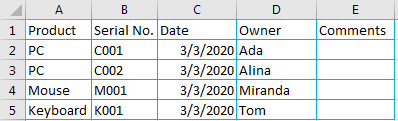Cells are separated by light blue line gridline. So let’s try to add this type of border for each cell automatically now.

## Add Borders Automatically by Conditional Formatting Function in Excel

Step 1: Select the range you want to create a table and apply the format for all cells. Refer to above table, we select the range A2:E5.

Step 2: Under Home tab, Styles frame, click Conditional Formatting ->New Rule.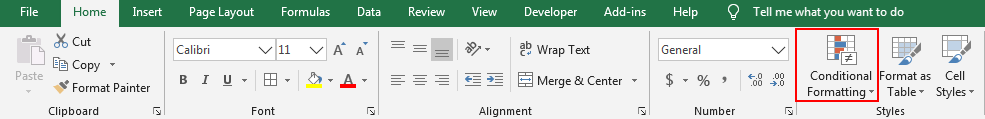Step 3: In New Formatting Rule window, select the last Rule Type ‘Use a formula to determine which cells to format’. Obviously, we need to add a formula to define a rule in Rule Description text box, and if this formula is true, the format values will automatically be applied for the cells satisfy the rule.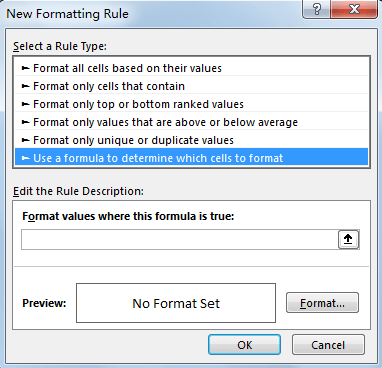Step 4: In ‘Format values where this formula is true:’ textbox, enter the formula =COUNTA(\$A\$1:\$E\$1)>0. In the formula, \$A\$1:\$E\$1 is the first row in the range for cells apply the format. COUNTA function returns the number of non-blank cell. So if we enter a value into any cell in \$A\$1:\$E\$1, a table with defined format will be created.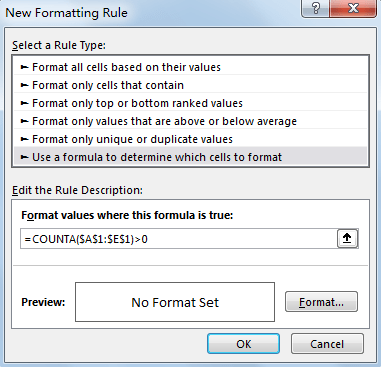Step 4: Click Format button just next to Preview. Verify that Format Cells window is loaded. Click on Border tab, select line style and color in Line frame, then click Outline in Presets, verify that you can see the preview in Border frame. Cell is applied with light blue gridline.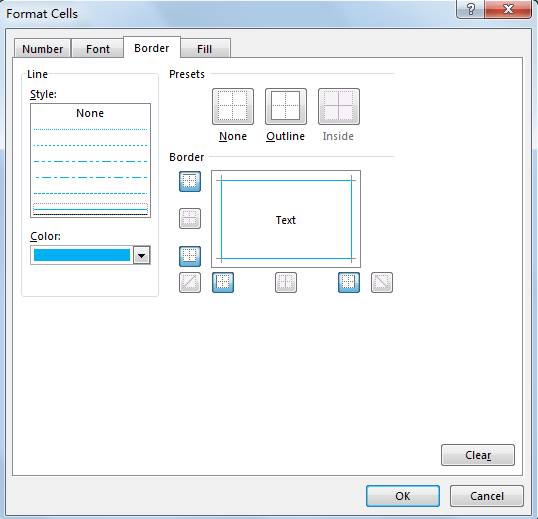Step 5: Click OK. Go back to New Formatting Rule window, verify that define format is displayed in Preview properly.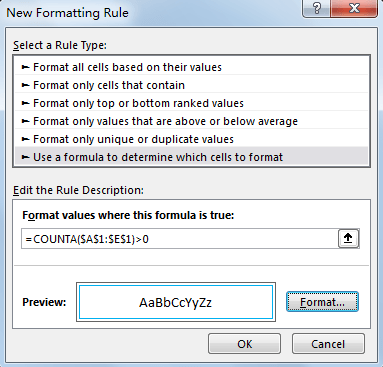Step 7: Select any cell in \$A\$1:\$E\$1, for example A1, enter a value ‘Product’.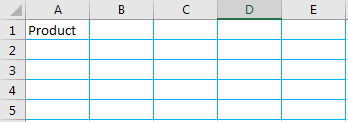Verify that format is applied for all cells in selected range properly.

Note:

1. Step1 is the key point, you must to select the selected range before using Conditional Formatting function. Otherwise this function doesn’t work.
2. In Formula you can type any formula as long as it can meet your requirement.

Related Posts

Calculate Years Between Dates In Ms Excel

If you are an avid Ms Excel user, then you might have come across a task in which you needed to calculate the years between the dates; you might take it easy and do this task manually, which is also ...

Calculate Number of Hours between Two Times

Calculating the difference between two times might be a valuable statistic for subsequent computations or averages, whether you're producing a time sheet for staff or recording personal exercises. While Excel has a plethora of complex functions, including date and time ...

Calculate Loan Interest in Given Year

When you borrow money, you are supposed to repay it gradually. Lenders, on the other hand, want to be compensated for their services and the risk they incur by lending you money. That is, you will not just repay the ...

Calculate Interest Rate for Loan

The interest rate is the fee charged by a lender to a borrower and is expressed as a percentage of the principal—the lent amount. The interest rate on a loan is often expressed as an annual percentage rate, abbreviated as ...

Calculate Interest for Given Period

Using the IPMT function in Excel, we can compute the interest payment on any loan. This step-by-step tutorial will guide Excel users of all skill levels through the process to calculate interest for given period. Finally, the formula: =IPMT(B3/12,1,B5,-B2) The ...

How To Use Excel GCD Function

This post will guide you how to use Excel GCD function with syntax and examples in Microsoft excel. Description The Excel GCD function Returns the greatest common divisor of two or more integers. So you can use the GCD function ...

Calculate A Ratio From Two Numbers In Excel

In elementary mathematics, a ratio is a connection or comparison between two or more integers. For example, ratios are often expressed as ":" to demonstrate the relationship between two numbers. You would think that manually calculating a ratio from two ...

How To Use Excel RRI Function

This post will guide you how to use Excel RRI function with syntax and examples in Microsoft excel. Description The Excel RRI function Returns an equivalent interest rate for the growth of an investment. So you can use the RRI ...

CAGR Formula Examples in Excel

CAGR in Excel is a formula that calculates the compound annual growth rate for any invested amount over the specified years or timeframe. Although there is no direct function in Excel that can help us identify the CAGR value, there ...

Build Hyperlink With VLOOKUP in Excel

You might have come across a task in which you were assigned to build hyperlinks, which seems very easy, and if you are new to excel or don't have enough experience with it, then you might wonder about doing this ...

Sidebar